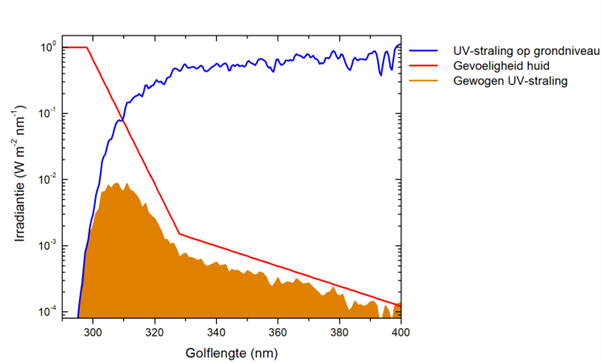RIVM measures the UV index continuously. On this page, we explain how we do this and which calculations are needed.

RIVM only measures the UV radiation that affects our health (UV-B and UV-A radiation). We do this every 12 minutes. We then use these data to calculate the UV index. This is done in a few internationally agreed steps.

1. We measure the strength of the UV-B and UV-A radiation in small increments for all relevant wavelengths (see the blue line in the figure below).
2. For each wavelength, we determine how sensitive the skin is to sunburn from UV radiation of that wavelength (see the red line in the figure below).
3. For each wavelength, we multiply the measured strength of the UV radiation by the sensitivity of the skin to becoming reddened. This is how the amount of UV radiation is weighted. The result of this multiplication gives us a new line (see the orange line in the figure below).
4. We calculate the size of the area under this orange line. In other words, we add up all weighted, measured UV strengths. This leaves us with just one number.
5. Because this number is awkwardly small, we then multiply it by 40. This is the internationally agreed multiplication factor. This results in a number that is usually between 0 and 10 in the Netherlands, but can be up to 15 elsewhere on earth. This number is called the UV index.
6. As a final step, we publish the UV index on our website and save it in a historical overview.## Measuring equipment

We measure UV radiation using two spectrophotometers located in Bilthoven. They perform highly accurate measurements of the amount of UV radiation in the range of 290–370 nm, in increments of 0.5 nm. The first spectrophotometer is a Dilor-XY, which has been in use since the 1990s. The second is a Brewer Mk III, which was commissioned in 2021. Each measurement takes 10 to 12 minutes.

We also use a few simple instruments (UVA meter, pyranometer) to improve the quality of the measurements further.

## Model

The UV radiation is not only measured, but also modelled. In the model, just like in real life, the UV radiation first passes through the ozone layer of the atmosphere. The percentage of each wavelength that passes through the ozone layer is calculated, depending on the thickness of the ozone layer at that time. It is then assumed that the remaining UV radiation passes through a layer of the atmosphere where there could be clouds. This reduces the UV radiation even further. The model thus calculates the percentage that ultimately reaches the ground.

To do so, however, the model needs accurate measurements of the thickness of the ozone layer and any clouds. The thickness of the ozone layer is measured by other institutes (Royal Netherlands Meteorological Institute, Royal Meteorological Institute of Belgium, Ozone Monitoring Instrument), using satellites and ground stations. Based on their weather models, they also provide forecasts of the thickness of the ozone layer for the coming days. This in turn allows us to forecast a few days in advance what the UV index would be if the sky remains clear.